# sklearn.metrics.silhouette_score¶

sklearn.metrics.silhouette_score(X, labels, metric=’euclidean’, sample_size=None, random_state=None, **kwds)[source]

Compute the mean Silhouette Coefficient of all samples.

The Silhouette Coefficient is calculated using the mean intra-cluster distance (a) and the mean nearest-cluster distance (b) for each sample. The Silhouette Coefficient for a sample is (b - a) / max(a, b). To clarify, b is the distance between a sample and the nearest cluster that the sample is not a part of. Note that Silhouette Coefficient is only defined if number of labels is 2 <= n_labels <= n_samples - 1.

This function returns the mean Silhouette Coefficient over all samples. To obtain the values for each sample, use silhouette_samples.

The best value is 1 and the worst value is -1. Values near 0 indicate overlapping clusters. Negative values generally indicate that a sample has been assigned to the wrong cluster, as a different cluster is more similar.

Read more in the User Guide.

Parameters: X : array [n_samples_a, n_samples_a] if metric == “precomputed”, or, [n_samples_a, n_features] otherwise Array of pairwise distances between samples, or a feature array. labels : array, shape = [n_samples] Predicted labels for each sample. metric : string, or callable The metric to use when calculating distance between instances in a feature array. If metric is a string, it must be one of the options allowed by metrics.pairwise.pairwise_distances. If X is the distance array itself, use metric="precomputed". sample_size : int or None The size of the sample to use when computing the Silhouette Coefficient on a random subset of the data. If sample_size is None, no sampling is used. random_state : int, RandomState instance or None, optional (default=None) The generator used to randomly select a subset of samples. If int, random_state is the seed used by the random number generator; If RandomState instance, random_state is the random number generator; If None, the random number generator is the RandomState instance used by np.random. Used when sample_size is not None. **kwds : optional keyword parameters Any further parameters are passed directly to the distance function. If using a scipy.spatial.distance metric, the parameters are still metric dependent. See the scipy docs for usage examples. silhouette : float Mean Silhouette Coefficient for all samples.

References

## Examples using sklearn.metrics.silhouette_score¶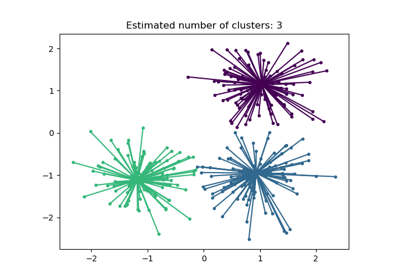Demo of affinity propagation clustering algorithm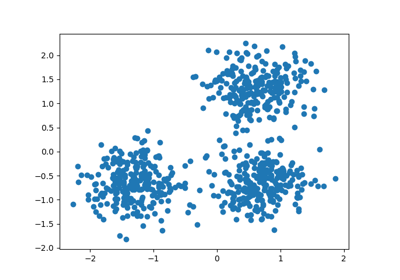Demo of DBSCAN clustering algorithm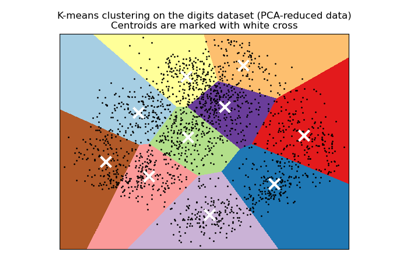A demo of K-Means clustering on the handwritten digits data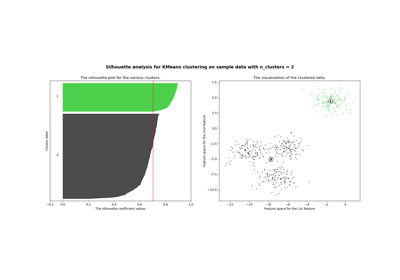Selecting the number of clusters with silhouette analysis on KMeans clustering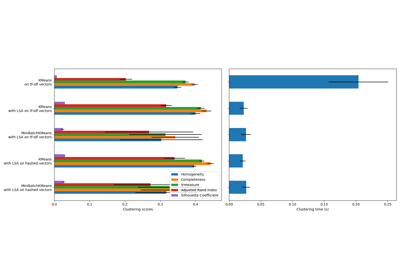Clustering text documents using k-means